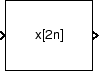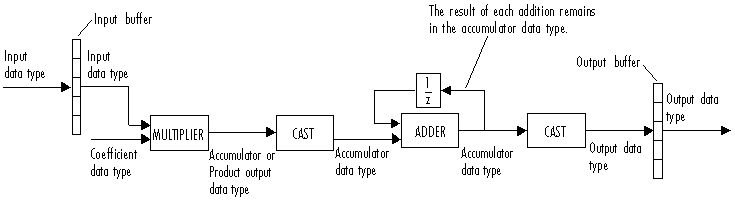# FIR Decimation

Filter and downsample input signals

## Library

Filtering / Multirate Filters

`dspmlti4`

•## Description

The FIR Decimation block resamples the discrete-time input at a rate K times slower than the input sample rate, where K is the integer value you specify for the Decimation factor parameter. To do so, the block implements a polyphase filter structure and performs the following operations:

1. Filters the data in each channel of the input using a direct-form FIR filter.

2. Downsamples each channel of filtered data by discarding K–1 consecutive samples following each sample that is retained.

The block uses a polyphase filter implementation because it is more efficient than straightforward filter-then-decimate algorithms. See Fliege  for more information.

You can use the FIR Decimation block inside triggered subsystems when you set the Rate options parameter to ```Enforce single-rate processing```.

This block supports variable-size input. This means that while the block is simulating, the frame size (number of rows) can change. The output dimensions always equal those of the input signal.

Under specific conditions, this block supports SIMD code generation. For details, see Code Generation.

### Specifying the Filter Coefficients

To specify the filter coefficients, select the mode you want the FIR Decimation block to operate in. Select the mode in the Coefficient source group box.

• Dialog parameters — Enter information about the filter, such as structure and coefficients, in the block dialog box.

• Input port — Specify the filter coefficients as an input to the block. Coefficient values are tunable (can change during simulation), while their properties must remain constant.

• Filter object — Specify the filter using a `dsp.FIRDecimator` System object™.

• Auto (default) — Choose the filter coefficients of an FIR Nyquist filter, predesigned for the decimation factor specified in the block dialog box.

When you select Dialog parameters, you use the FIR filter coefficients parameter to specify the numerator coefficients of the FIR filter transfer function H(z).

`$H\left(z\right)=B\left(z\right)={b}_{1}+{b}_{2}{z}^{-1}+\dots +{b}_{m}{z}^{-\left(m-1\right)}$`

You can generate the FIR filter coefficient vector, ```[b(1) b(2) ... b(m)]```, using one of the DSP System Toolbox™ filter design functions such as `designMultirateFIR`, `firnyquist`, `firhalfband`, `firgr`, or `firceqrip`.

The filter you specify must be a lowpass filter with a normalized cutoff frequency no greater than 1/K. The block internally initializes all filter states to zero.

When you select Auto, the block designs an FIR decimator with the decimation factor specified in Decimation factor. The `designMultirateFIR` function designs the filter and returns the coefficients used by the block. For more information on the filter design, see Orfanidis .

### Frame-Based Processing

When you set the Input processing parameter to `Columns as channels (frame based)`, the block resamples each column of the input over time. In this mode, the block can perform either single-rate or multirate processing. You can use the Rate options parameter to specify how the block resamples the input:

• When you set the Rate options parameter to `Enforce single-rate processing`, the input and output of the block have the same sample rate. To decimate the output while maintaining the input sample rate, the block resamples the data in each column of the input such that the frame size of the output (Mo) is 1/K times that of the input (Mo = Mi/K),

In this mode, the input frame size, Mi, must be a multiple of the Decimation factor, K.

For an example of single-rate FIR Decimation, see Example 1 — Single-Rate Processing.

• When you set the Rate options parameter to `Allow multirate processing`, the input and output of the FIR Decimation block are the same size, but the sample rate of the output is K times slower than that of the input. In this mode, the block treats an Mi-by-N matrix input as N independent channels. The block decimates each column of the input over time by keeping the frame size constant (Mi=Mo), and making the output frame period (Tfo) K times longer than the input frame period (Tfo = K*Tfi).

See Example 2— Multirate Frame-Based Processing for an example that uses the FIR Decimation block in this mode.

### Sample-Based Processing

When you set the Input processing parameter to `Elements as channels (sample based)`, the block treats an M-by-N matrix input as M*N independent channels, and decimates each channel over time. The output sample period (Tso) is K times longer than the input sample period (Tso = K*Tsi), and the input and output sizes are identical.

### Latency

When you use the FIR Decimation block in sample-based processing mode, the block always has zero-tasking latency. Zero-tasking latency means that the block propagates the first filtered input sample (received at time t= 0) as the first output sample. That first output sample is then followed by filtered input samples K+1, 2K+1, and so on.

When you use the FIR Decimation block in frame-based processing mode with a frame size greater than one, the block may exhibit one-frame latency. Cases of one-frame latency can occur when the input frame size is greater than one, and you set the Input processing and Rate options parameters of the FIR Decimation block as follows:

• Input processing = ```Columns as channels (frame based)```

• Rate options = ```Allow multirate processing```

In cases of one-frame latency, you can define the value of the first Mi output rows by setting the Output buffer initial conditions parameter. The default value of the Output buffer initial conditions parameter is `0`. However, you can enter a matrix containing one value for each channel of the input, or a scalar value to be applied to all channels. The first filtered input sample (first filtered row of the input matrix) appears in the output as sample Mi+ 1. That sample is then followed by filtered input samples K+ 1, 2K+ 1, and so on.

### Fixed-Point Data Types

The following diagram shows the data types used within the FIR Decimation block for fixed-point signals.You can set the coefficient, product output, accumulator, and output data types in the block dialog box as discussed in the Dialog Box section. This diagram shows that data is stored in the input buffer with the same data type and scaling as the input. The block stores filtered data and any initial conditions in the output buffer using the output data type and scaling that you set in the block dialog box.

When at least one of the inputs to the multiplier is real, the output of the multiplier is in the product output data type. When both inputs to the multiplier are complex, the result of the multiplication is in the accumulator data type. For details on the complex multiplication performed by this block, see Multiplication Data Types.

### Note

When the block input is fixed point, all internal data types are signed fixed-point values.

## Examples

### Example 1 — Single-Rate Processing

In the ex_firdecimation_ref2 model, the FIR Decimation block decimates a single-channel input with a frame size of 64. Because the block is doing single-rate processing and the Decimation factor parameter is set to `4`, the output of the FIR Decimation block has a frame size of 16. As shown in the following figure, the input, and output of the FIR Decimation block have the same sample rate.### Example 2— Multirate Frame-Based Processing

In the ex_firdecimation_ref1 model, the FIR Decimation block decimates a single-channel input with a frame period of one second. Because the block is doing multirate frame-based processing and the Decimation factor parameter is set to `4`, the frame period of the output is `4` seconds. As shown in the following figure, the input, and output of the FIR Decimation block have the same frame size, but the sample rate of the output is four times that of the input.### Example 3

The `ex_polyphasedec` model illustrates the underlying polyphase implementations of the FIR Decimation block. Run the model, and view the results on the scope. The output of the FIR Decimation block matches the output of the Polyphase Decimation Filter block.

### Example 4

The `ex_mrf_nlp` model illustrates the use of the FIR Decimation block in several multistage multirate filters.

## Dialog Box

### Coefficient Source

The FIR Decimation block can operate in four different modes. Select the mode in the Coefficient source group box.

• Dialog parameters — Enter information about the filter, such as structure and coefficients, in the block mask.

• Input port — Specify the filter coefficients with a Num input port. The Num input port appears when you select the Input port option. Coefficient values obtained through Num are tunable (can change during simulation), while their properties must remain constant.

• Filter object — Specify the filter using a `dsp.FIRDecimator` System object.

• Auto (default) — Choose the coefficients of an FIR Nyquist filter, predesigned for the decimation factor specified in the block dialog box.

Different items appear on the FIR Decimation block dialog box depending on whether you select Dialog parameters, Input port, Filter object, or Auto in the Coefficient source group box.

## References

 Fliege, N. J. Multirate Digital Signal Processing: Multirate Systems, Filter Banks, Wavelets. West Sussex, England: John Wiley & Sons, 1994.

 Orfanidis, Sophocles J. Introduction to Signal Processing. Upper Saddle River, NJ: Prentice-Hall, 1996.

## Supported Data Types

PortSupported Data Types

Input

• Double-precision floating point

• Single-precision floating point

• Fixed point

• 8-, 16-, and 32-bit signed integers

• 8-, 16-, and 32-bit unsigned integers

Output

• Double-precision floating point

• Single-precision floating point

• Fixed point

• 8-, 16-, and 32-bit signed integers

• 8-, 16-, and 32-bit unsigned integers

Watch now